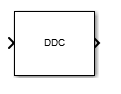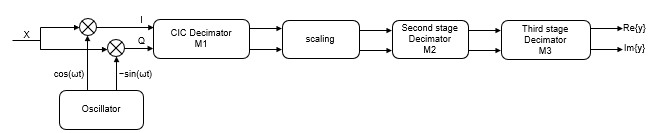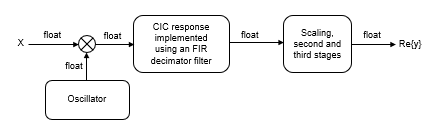# Digital Down-Converter

Translate digital signal from intermediate frequency (IF) band to baseband and decimate it

•Libraries:
DSP System Toolbox / Signal Operations

## Description

The Digital Down-Converter (DDC) block converts a digitized real signal centered at an intermediate frequency (IF) to a baseband complex signal centered at zero frequency. The DDC block downsamples the frequency downconverted signal using a cascade of three decimation filters. This block designs the decimation filters according to the filter parameters you set in the block dialog box.

## Ports

### Input

expand all

Specify the data input as a column vector or a matrix. The number of rows in the input signal must be a multiple of the decimation factor that you specify in the Decimation factor parameter.

When the input data type is `double` or `single` precision, the output data type is the same as that of the input. When the input data type is a signed integer or fixed-point type, the output data type is defined by the Output parameter on the Data Types tab.

When the input is fixed-point, it must be signed only.

Data Types: `single` | `double` | `int8` | `int16` | `int32` | `int64` | `fixed point`
Complex Number Support: Yes

Specify the oscillator signal that the block uses to frequency down convert the input signal as a column vector or a matrix. The signal must be complex and its length must be equal to the length of the input.

When the oscillator input is fixed-point, it must be signed only.

#### Dependencies

To enable this port, set the Type of oscillator parameter to `Input port`.

Data Types: `single` | `double` | `int8` | `int16` | `int32` | `int64` | `fixed point`
Complex Number Support: Yes

### Output

expand all

The block outputs the downconverted and downsampled signal as a column vector or a matrix. The length of the output is equal to the length of the input divided by the value you specify in the Decimation factor parameter.

When the data type of the input is `double` or `single` precision, the data type of the output is the same as that of the input. When the input data type is fixed-point type or a signed integer, the output data type is defined by the Output parameter on the Data Types tab.

Data Types: `single` | `double` | `int8` | `int16` | `int32` | `int64` | `fixed point`
Complex Number Support: Yes

## Parameters

expand all

### Main Tab

Specify the decimation factor as a positive integer ≥ 2, or as a 1-by-2 or 1-by-3 vector of positive integers.

When you set this parameter to a scalar value, the block applies the same decimation factor to the three decimation filtering stages.

When you set this parameter to a 1-by-2 vector, the block applies the first and second values in the vector to the first and second filtering stages, respectively, and bypasses the third stage. Both elements of Decimation factor must be greater than 1.

When you set this parameter to a 1-by-3 vector, the block applies the values in the vector to the corresponding filtering stages. The first and second elements of Decimation factor must be greater than 1, and the third element must be 1 or 2.

When you select this check box, the block designs filters with the minimum possible order that meets the requirements specified in these parameters:

• Passband ripple of cascade response (dB)

• Stopband attenuation of cascade response (dB)

• Two sided bandwidth of input signal (Hz)

• Source of stopband frequency

• Stopband frequency (Hz)

When you clear this check box, the block designs filters with orders that you specify in Number of sections of CIC decimator, Order of CIC compensation filter stage, and Order of third filter stage. The filter designs meet the passband and stopband frequency specifications that you set in Two sided bandwidth of input signal (Hz), Source of stopband frequency, and Stopband frequency (Hz). By default, this check box is selected.

Specify the number of sections in the CIC decimator as a positive integer.

#### Dependencies

To enable this parameter, clear the Minimum order filter design parameter.

Specify the order of the third filter stage as an even positive integer. When you specify Decimation factor as a 1-by-2 vector, the block ignores the value in this parameter because the block bypasses the third filter stage.

#### Dependencies

To enable this parameter, clear the Minimum order filter design parameter.

Specify the source of the stopband frequency as `Auto` or `Property`.

When you set this parameter to `Auto`, the block places the cutoff frequency of the cascade filter response at approximately Fc = SampleRate / M/2 Hz, where M is the total decimation factor specified in Decimation factor. SampleRate is computed as `1`/ Ts, where Ts is the sample time of the input signal. The block computes the stopband frequency as Fstop = Fc + (TW / 2). TW is the transition bandwidth of the cascade response, computed as 2×(FcFp), where the passband frequency Fp equals Bandwidth/2.

When you set this parameter to `Property`, specify the stopband frequency in Stopband frequency (Hz).

Specify the oscillator type as one of the following:

• `Sine wave` (default) — The block performs frequency down conversion on the input signal using a complex exponential obtained from samples of a sinusoidal trigonometric function.

• `NCO` — The block performs frequency down conversion on the input signal with a complex exponential obtained using a numerically controlled oscillator (NCO).

• `Input port` — The block performs frequency down conversion on the input signal using the complex signal that you provide through the Osc input port.

• `None` — The block does not require the mixer stage and acts as a three-stage cascaded decimator.

Specify the center frequency of the output signal in Hz as a double-precision positive scalar that is less than or equal to half the sample rate. The block downconverts the input signal from the passband center frequency, which you specify in Center frequency of input signal (Hz), to `0` Hz.

#### Dependency

To enable this parameter, set Type of oscillator to `Sine wave` or `NCO`.

Specify the order of the CIC compensation filter stage as a positive integer.

#### Dependencies

To enable this parameter, clear the Minimum order filter design parameter.

Specify the two-sided bandwidth of the input signal in Hz as a positive integer. The block sets the passband frequency of the cascade of filters to half of the value you specify in this parameter. Set the value of this parameter to less than Input sample rate/Decimation factor. When you select the Inherit sample rate from input check box, set this value to less than ((`1`/Ts) / Decimation factor), where Ts is the sample time of the input signal.

Specify the stopband frequency in Hz as a double-precision positive scalar.

#### Dependencies

To enable this parameter, set Source of stopband frequency to `Property`.

Specify the passband ripple of the cascade response in dB as a double-precision positive scalar. When you select the Minimum order filter design, the block designs the filters so that the cascade response meets the passband ripple that you specify in Passband ripple of cascade response (dB).

#### Dependencies

To enable this parameter, select the Minimum order filter design parameter.

Specify the stopband attenuation of the cascade response in dB as a double-precision positive scalar. When you select the Minimum order filter design parameter, the block designs the filters such that the cascade response meets the stopband attenuation that you specify in this parameter.

#### Dependencies

To enable this parameter, select the Minimum order filter design parameter.

Specify the number of NCO accumulator bits as an integer scalar in the range [`1 128`].

#### Dependencies

To enable this parameter, set Type of oscillator to `NCO`.

Specify the number of NCO quantized accumulator bits as an integer scalar in the range `[1 128]`. This value must be less than the value you specify in Number of NCO accumulator bits.

#### Dependencies

To enable this parameter, set Type of oscillator to `NCO`.

When you select this check box, the block applies dither to the NCO signal according to the number of dither bits you specify in Number of NCO dither bits. By default, this check box is selected.

#### Dependencies

To enable this parameter, set Type of oscillator to `NCO`.

Specify the number of NCO dither bits as an integer scalar smaller than the number of accumulator bits in Number of NCO accumulator bits.

#### Dependencies

To enable this parameter, set Type of oscillator to `NCO` and select the Dither control for NCO parameter.

When you select this check box, the block computes the sample rate as `N`/Ts, where N is the frame size of the input signal, and Ts is the sample time of the input signal. When you clear this check box, the block sets the sample rate to the value in Input sample rate (Hz).

Specify the sample rate of the input signal in Hz as a positive scalar value greater than or equal to twice the value in Center frequency of input signal (Hz).

#### Dependencies

To enable this parameter, clear the Inherit sample rate from input parameter.

Click this button to open the Filter Visualization Tool (FVTool) and display the magnitude and phase response of each stage as well as the cascade of stages in the Digital Down-Converter. The response is based on the values you specify in the block parameters dialog box. Changes made to these parameters update FVTool.To update the magnitude response while FVTool is running, modify the parameters in the dialog box and click .

Specify the type of simulation to run. You can set this parameter to:

• `Code generation` (default)

Simulate model using generated C code. The first time you run a simulation, Simulink® generates C code for the block. The C code is reused for subsequent simulations, as long as the model does not change. This option requires additional startup time but provides faster simulation speed than ```Interpreted execution```.

• `Interpreted execution`

Simulate model using the MATLAB®  interpreter. This option shortens startup time but has slower simulation speed than ```Code generation```.

### Data Types Tab

Specify the data type of the input in the first, second, and third filter stages. You can set this parameter to one of the following:

• `Inherit: Same as input` (default) — The block inherits the Stage input data type from the input signal.

• `fixdt([],16,0)` — The block uses the fixed-point data type with binary-point scaling. Specify the sign mode of this data type as `[]` or `true`.

• An expression that evaluates to a data type, for example, `numerictype([],16,15)`. Specify the sign mode of this data type as `[]` or `true`.

For help with setting the stage input parameter, you can click the buttonto display the data type assistant.

Specify the data type of the block output. You can set this parameter to:

• `Inherit: Same as input` (default) — The block Inherits the output datatype from the input.

• `fixdt([],16,0)` — The block uses the fixed-point data type with binary point scaling. Specify the sign mode of this data type as `[]` or `true`.

• An expression that evaluates to a data type, for example, `numerictype([],16,15)`. Specify the sign mode of this data type as `[]` or `true`.

For help with setting the Output parameter, you can click the buttonto display the data type assistant.

Specify the minimum value of the block output. The default value is `[]` (unspecified). Simulink software uses this value to perform:

• Simulation range checking (see Specify Signal Ranges (Simulink))

• Automatic scaling of fixed-point data types

Maximum value of the block output. The default value is `[]` (unspecified). Simulink software uses this value to perform:

• Simulation range checking (see Specify Signal Ranges (Simulink))

• Automatic scaling of fixed-point data types

Select this parameter to prevent the fixed-point tools from overriding the data types you specify in the block dialog box.

## Block Characteristics

 Data Types `double` | `fixed point` | `integer` | `single` Direct Feedthrough `no` Multidimensional Signals `no` Variable-Size Signals `no` Zero-Crossing Detection `no`

expand all

## Algorithms

The digital down converter downconverts the input signal by multiplying it with a complex exponential that has the specified center frequency. The algorithm downsamples the frequency downconverted signal using a cascade of three decimation filters. In this case, the filter cascade consists of a CIC decimator, a CIC compensator, and a third FIR decimation stage. The following block diagram shows the architecture of the digital down converter.The scaling section normalizes the CIC gain and the oscillator power. It can also contain a correction factor to achieve the desired ripple specification. When you specify an oscillator signal through the input port, the normalization factor does not include the oscillator power factor. Depending on how you set the decimation factor, the block bypasses the third filter stage. When the input data type is double or single, the algorithm implements an N-section CIC decimation filter as an FIR filter with a response that corresponds to a cascade of N boxcar filters. The algorithm emulates a CIC filter with an FIR filter so that you can run simulations with floating-point data. When the input data type is fixed-point, the algorithm implements a true CIC filter with actual comb and integrator sections.

This block diagram represents the DDC arithmetic with single or double-precision, floating-point inputs.For details about fixed-point operation, see Fixed Point.

## Version History

Introduced in R2015a